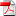# Option valuation and hedging using asymmetric risk function: asymptotic optimality through fully nonlinear Partial Differential Equations - Emmanuel Gobet, Isaque Pimentel, Xavier Warin

18
Jun

Discrete time hedging produces a residual risk, namely, the tracking error. The major problem is to get valuation/hedging policies minimizing this error. We evaluate the risk between trading dates through a function penalizing asymmetrically profits and losses. After deriving the asymptotics within a discrete time risk
measurement for a large number of trading dates, we derive the optimal strategies minimizing the asymptotic risk in the continuous time setting. We characterize the optimality through a class of fully nonlinear Partial Differential Equations (PDE). Numerical experiments show that the optimal strategies associated with discrete
and asymptotic approach coincides asymptotically.

Keywords: hedging; asymmetric risk; fully nonlinear parabolic PDE; regression
Monte Carlo.

•RR-FIME-18-01